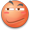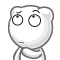• 啊，好久没更新过博客了~~
• 余式定理相关题目中，总有一种比较抽象的题型会比较讨厌。相对来说难以理解：把过程记下来了不用一段时间就会忘却，尝试去理解重新推导成本很高。
• 为了防止我以后忘记这个思路，也为了防止我手贱丢掉那张草稿纸，我决定把这个思路记下来。

## 例题

• 已知 $$f\left(x\right)=A\times\left(x-1\right)+2$$ 除以 $$x-1$$ 余式为 $$1$$ ; 除以 $$x^{2}-2x+3$$ 余式为 $$4x+6$$ ;
求 $$f\left( x\right)$$ 除以 $$\left( x-1\right) \left( x^{2}-2x+3\right)$$ 的余式。

## 解

• 由题得两式子
• $$f\left(x\right)=A\times\left(x-1\right)+2$$ ①
• $$f\left(x\right)=B\times\left(x^{2}-2x+3\right)+4x+6$$ ②
• $$f\left(x\right)=C\times\left(x-1\right)\left(x^{2}-2x+3\right) + ax^{2}+bx+c$$ ③ ← 顺便设一个式子
• 因为 $$f\left( x\right)$$ 可以由上面的随便一个式子来表达，所以我们就拿我们设出来的③式来除上面任意的一个式子。当然，为了方便处理，一般都除以除式次数较高的那一条。
• 操作一下 $$f\left(x\right)=C\times\left(x-1\right)\left(x^{2}-2x+3\right) + ax^{2}+bx+c$$ 除以 $$x^{2}-2x+3$$ 得到 $$f\left(x\right)=\left(x^{2}-2x+3\right)\times\left(C\times\left(x-1\right)+a\right)+\left(\left(b+2a\right)x+\left(c-3a\right)\right)$$
因为上面得出的式子是相当于由 $$f\left( x\right)$$ 除以 $$x^{2}-2x+3$$ 得出的。所以这条式子明显等价于 ②式。
• 我们对其"待定系数法"一下，得到两个等式

1. $$b+2a=4$$
2. $$c-3a=6$$
• 啊，这不对吧？两个式子怎么可以求出三个未知数？
我们回到题目看一下：我们由始至终还有①式子未使用。 最后我们再加一个 $$f\left( 1\right)=2$$ 也就是 $$a+b+c=2$$ 来限定 $$a$$,$$b$$,$$c$$之间的关系就可以了。
• 最后，得出来的方程组有三条等式。分别是：

1. $$b+2a=4$$
2. $$c-3a=6$$
3. $$a+b+c=2$$
• 解出来之后， $$a=-4$$ $$b=12$$ $$c=-6$$
• 即 $$f\left( x\right)$$ 除以 $$\left( x-1\right) \left( x^{2}-2x+3\right)$$ 的余式 $$-4x^{2}+12x-6$$

## 一些话

• 题目来源 : 为啥我算的答案和上面网友给出来的答案不一样，却和小猿搜题给出的答案一样• 其实我文中的方法，在高二的时候老师讲过了。但是，不知道为啥，一直迷迷糊糊的，理解不是很透彻，知道我高三的上数学课复习的时候才想清楚到底这个方法是为什么。
• 为了想清楚这个方法到底是为什么，好像、、、好像那节数学课、、、我没听课、、、、• 写这篇文章一不小心就花了我近一个小时，感觉挺值得的。对于我来说，我也顺便回顾了一下这个算法。以防下次考试出现这个题型的时候再次懵逼。